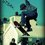# Prove U can't get an constant.

Let us make a series. $S=1+\frac{1}{2}+\frac{1}{3}+\frac{1}{4}+\frac{1}{5}+\frac{1}{6}..............$ Prove that this will not converge to a constant.

Extra Credit: Do it without use of calculus.Note by Utsav Singhal
5 years, 10 months ago

This discussion board is a place to discuss our Daily Challenges and the math and science related to those challenges. Explanations are more than just a solution — they should explain the steps and thinking strategies that you used to obtain the solution. Comments should further the discussion of math and science.

When posting on Brilliant:

• Use the emojis to react to an explanation, whether you're congratulating a job well done , or just really confused .
• Ask specific questions about the challenge or the steps in somebody's explanation. Well-posed questions can add a lot to the discussion, but posting "I don't understand!" doesn't help anyone.
• Try to contribute something new to the discussion, whether it is an extension, generalization or other idea related to the challenge.

MarkdownAppears as
*italics* or _italics_ italics
**bold** or __bold__ bold
- bulleted- list
• bulleted
• list
1. numbered2. list
1. numbered
2. list
Note: you must add a full line of space before and after lists for them to show up correctly
paragraph 1paragraph 2

paragraph 1

paragraph 2

[example link](https://brilliant.org)example link
> This is a quote
This is a quote
    # I indented these lines
# 4 spaces, and now they show
# up as a code block.

print "hello world"
# I indented these lines
# 4 spaces, and now they show
# up as a code block.

print "hello world"
MathAppears as
Remember to wrap math in $$ ... $$ or $ ... $ to ensure proper formatting.
2 \times 3 $2 \times 3$
2^{34} $2^{34}$
a_{i-1} $a_{i-1}$
\frac{2}{3} $\frac{2}{3}$
\sqrt{2} $\sqrt{2}$
\sum_{i=1}^3 $\sum_{i=1}^3$
\sin \theta $\sin \theta$
\boxed{123} $\boxed{123}$

Sort by:

The proof that I like is by contradiction.

Suppose $S$ converges to a finite number. Then, show that $S = S + 1$.

(details to follow).

Staff - 5 years, 8 months ago

Even though the harmonic series does not converge, Is it possible for a partial sum of the series to be an integer?

- 5 years, 8 months ago

Let us make a AM HM inequality. 1/(a-1) + 1/a + 1/(a+1) >= 3/a So we will take its minnimum value... as 3/a Now lets put the values . Leaving 1 then taking 1/2 , 1/3, 1/4 then next three....then next three... So we will get its min value as 1 + 1 + 1/2 + 1/3. ..... to infinity. Then again doing it. 1+1+1+1+1+1+..................to infinite +1/2 + 1/3 + 1/4 .... So its minnimum value comes out to be infinite .

Soorry can't make the solution latex ...!

- 5 years, 10 months ago

This is popularly known as the divergence of the Harmonic Series- 5 years, 10 months ago

It can be done using RATIO TEST.

- 5 years, 10 months ago

Please give the details or any link from where I can learn it.. Thanks.

- 5 years, 10 months ago

- 5 years, 10 months ago

But the limit L here is 1. Therefore, test becomes inconclusive

- 5 years, 8 months ago

2.30 approx

- 5 years, 10 months ago

No no, it is totally wrong . There is no value of it . It will be infinite

- 5 years, 10 months ago